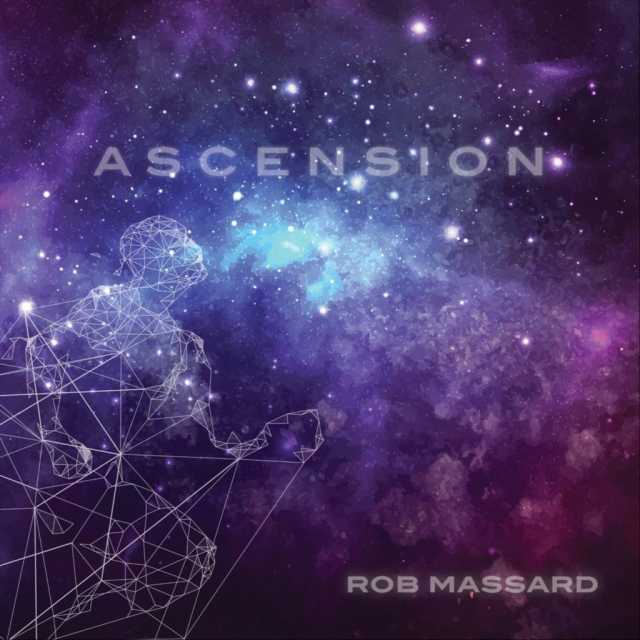Art, History, Music, Politics, and Spirituality For The Modern Alchemist – circulation in over 129 countries

# The Sign of Sheba Year 18,002: Begins June 28th 2014

There are eight months in the Calendar of Mu, sometimes referred to as the Nyarzirian Calendar. Each month consists of forty-five days, and represents a system of the eight hexagrams moving through the five elements. The Ninzuwu Priest/Priestess invokes the mudra/mantra formulae associated with each hexagram that rules a particular day after invoking the Armor of Amaterasu Ohkami. The chart below illustrates the daily Vasuh letters invoked and the ruling “deity” for the said hexagram:

1. June 28th, 2014 = Tasuta = 9th Hexagram = Hmu-Bnhu
2. June 29th 2014 = Kukunochi = 20th Hexagram = Hmu-Tuu
3. June 30th 2014 = Ieh = 37th Hexagram = Zhee-Zhee-Lewhu
4. July 1st 2014 = Gzk = 42nd Hexagram = Zhee-Tuu-Hmu-Nzu-Aum
5. July 2nd 2014 = Jhn = 53rd Hexagram = Zhee-Bnhu
6. July 3rd 2014 = Sheba = 57th Hexagram = Hmu-Shki-Bnhu-Bnhu
7. July 4th 2014 = Lzwa = 59th Hexagram = Aum-Shki
8. July 5th 2014 = Kuhvi = 61st Hexagram = Phe-Phe
9. July 6th 2014 =Entering Earth Element
10. July 7th 2014 = Tasuta = 9th Hexagram = Hmu-Bnhu
11. July 8th 2014 = Kukunochi = 20th Hexagram = Hmu-Tuu
12. July 9th 2014 = Ieh = 37th Hexagram = Zhee-Zhee-Lewhu
13. July 10th 2014 = Gzk = 42nd Hexagram = Zhee-Tuu-Hmu-Nzu-Aum
14. July 11th 2014 = Jhn = 53rd Hexagram = Zhee-Bnhu
15. July 12th 2014 = Sheba = 57th Hexagram = Hmu-Shki-Bnhu-Bnhu
16. July 13th 2014 = Lzwa = 59th Hexagram = Aum-Shki
17. July 14th 2014 = Kuhvi = 61st Hexagram = Phe-Phe
18. July 15th 2014 =Entering Water Element
19. July 16th 2014 = Tasuta = 9th Hexagram = Hmu-Bnhu
20. July 17th 2014 = Kukunochi = 20th Hexagram = Hmu-Tuu
21. July 18th 2014 = Ieh = 37th Hexagram = Zhee-Zhee-Lewhu
22. July 19th 2014 = Gzk = 42nd Hexagram = Zhee-Tuu-Hmu-Nzu-Aum
23. July 20th 2014 = Jhn = 53rd Hexagram = Zhee-Bnhu
24. July 21st 2014 = Sheba = 57th Hexagram = Hmu-Shki-Bnhu-Bnhu
25. July 22nd 2014 = Lzwa = 59th Hexagram = Aum-Shki
26. July 23rd 2014 = Kuhvi = 61st Hexagram = Phe-Phe
27. July 24th 2014 =Entering Fire Element
28. July 25th 2014 = Tasuta = 9th Hexagram = Hmu-Bnhu
29. July 26th 2014 = Kukunochi = 20th Hexagram = Hmu-Tuu
30. July 27th 2014 = Ieh = 37th Hexagram = Zhee-Zhee-Lewhu
31. July 28th 2014 = Gzk = 42nd Hexagram = Zhee-Tuu-Hmu-Nzu-Aum
32. July 29th 2014 = Jhn = 53rd Hexagram = Zhee-Bnhu
33. July 30th 2014 = Sheba = 57th Hexagram = Hmu-Shki-Bnhu-Bnhu
34. July 31st 2014 = Lzwa = 59th Hexagram = Aum-Shki
35. August 1st 2014 = Kuhvi = 61st Hexagram = Phe-Phe
36. August 2nd 2014 =Entering Air Element
37. August 3rd 2014 = Tasuta = 9th Hexagram = Hmu-Bnhu
38. August 4th 2014 = Kukunochi = 20th Hexagram = Hmu-Tuu
39. August 5th 2014 = Ieh = 37th Hexagram = Zhee-Zhee-Lewhu
40. August 6th 2014 = Gzk = 42nd Hexagram = Zhee-Tuu-Hmu-Nzu-Aum
41. August 7th 2014 = Jhn = 53rd Hexagram = Zhee-Bnhu
42. August 8th 2014 = Sheba = 57th Hexagram = Hmu-Shki-Bnhu-Bnhu
43. August 9th 2014 = Lzwa = 59th Hexagram = Aum-Shki
44. August 10th 2014 = Kuhvi = 61st Hexagram = Phe-Phe
45. August 11th 2014 =Entering Void Element

#### You may have missed#### October by Mae Simpson#### Night Owl by Adrian John Szozda